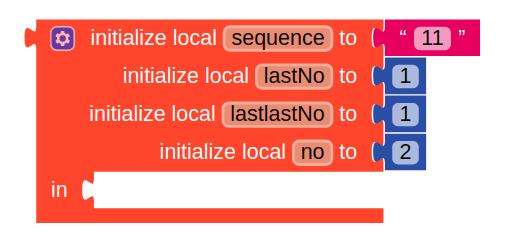# Daily Challenge #55

The Fibonacci sequence is the series of numbers: 1, 1, 2, 3, 5, 8, 13, 21, 34, … The next number is found by adding up the two numbers before it. (source)
Your task is to ask for a number and the program should return the digit of the series.

Sample imputs:

``````fibonacci(8) => 3 #1, 1, 2, 3, 5, 8, 1𝟯,...
fibonacci(18) => 4
fibonacci(24) => 7
fibonacci(234) => 3
fibonacci(1834) => 5
``````

Have fun!

btw, start the sequence from 1 instead from 0.

1 Like

This is a variation of

Great to see the daily challenges again. Thanks @Mateja

2 Likes

I will be posting my solution here, but if you want to solve this I suggest not opening the answer.

Solution

fibonacciSequence/src-py at master · ontstudios/fibonacciSequence · GitHub

1 Like

Hi @Mateja,

a little tip. The initialize local block is a mutator block. Any block with the blue gear sign is one.